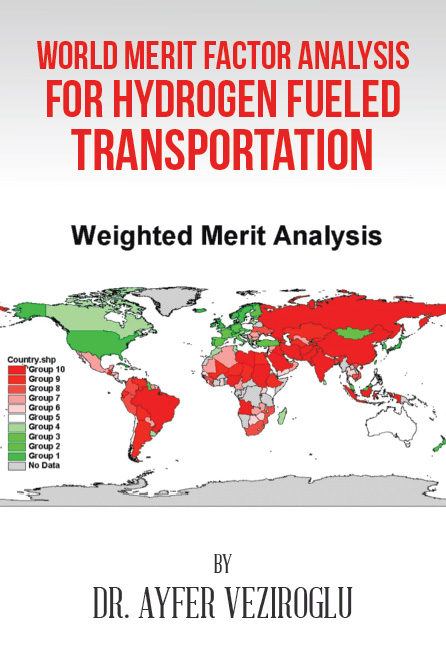Books World Merit Factor Analysis for Hydrogen Fueled Transportation

# World Merit Factor Analysis for Hydrogen Fueled TransportationWorld Merit Factor Analysis for Hydrogen Fueled Transportation covers almost all the countries of the world in order to decide which countries would be able to convert to hydrogen-fueled transportation earlier and which countries would do it later.

We have decided that the following nine factors (which will be called “influence factors”) will have more impact for a country’s conversion to hydrogen-fueled transportation than other factors:

—Size
—Population
—Income per capita
—Educational level
—Borders
—Petroleum dependence
—Vehicles per capita
—Hydrogen-filling stations

We have obtained the data for size (S), population (P), income per capita (I), and vehicles per thousand of population (V) for the year 2008. We have calculated educational expenditures as percentage of GDP for education level (E) for 2007.

After the basic merit factor analysis, we assumed that all the dimensionless influence factors have the same effect on the merit factors for deciding whether a country could move to the hydrogen-fueled transportation system faster than the other countries. In other words, we assumed that each dimensionless influence factor has a weight of one.

In the real world, some influence factors will have a greater effect than the others. Then we defined a new weighted merit factor. We have developed an analytical method in order to access the potential of countries for conversion to hydrogen-fueled transportation, which we have named “merit factor analysis.” This has been based on the selection of influence factors, which influence the outcome, and on the decision of whether the effect of a given influence factor will support the desired outcome or will be an obstacle to the desired outcome. We have further refined our analytical system by the introduction of weighting factors that measure the impact of a given influence factor for the desired outcome or against the desired outcome. We have named the two versions of the analytical method “basic merit factor analysis” and “weighted merit factor analysis” respectively.

Of course, the analytical method that we have developed could be applied to other cases in order to compare the potential of a given entity for a desired outcome. For example, it could be applied for the profit potentials of commercial companies by selecting the appropriate influence factors and weighting factors.

Click to purchase